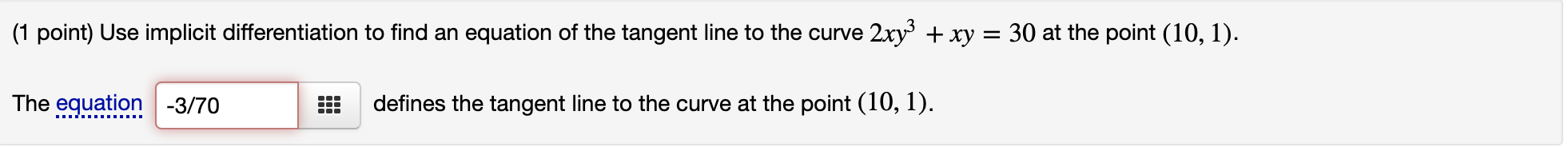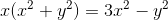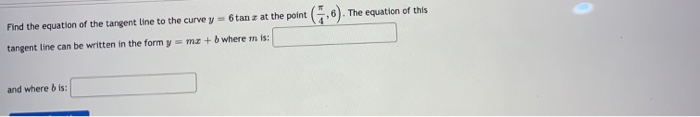Question

# Use implicit differentiation to find the equation of the tangent line to the curve xy^3+xy=5 at the point (4,1)

Use implicit differentiation to find the equation of the tangent line to the curve xy^3+xy=5 at the point (4,1) . The equation of this tangent line can be written in the form y = mx+b where m is:?
and where b is:?

ok i got result for m. but i dont know where is b.. i forget how do y=mx+b
see other post.
hey mily how u get result for m?

#### Earn Coins

Coins can be redeemed for fabulous gifts.

Similar Homework Help Questions
• ### Use implicit differentiation to find the equation of the tangent line to the curve xy^3+2xy=9 at the point (31)

Use implicit differentiation to find the equation of the tangent line to the curve xy^3+2xy=9 at the point (31). The equation of this tangent line can be written in the form y=mx+b where m is

• ### Use implicit differentiation to find the equation of the tangent line to the curve xy3+xy=14 at the point (7,1)

Use implicit differentiation to find the equation of the tangent line to the curve xy3+xy=14 at the point (7,1) . The equation of this tangent line can be written in the form y=mx+bi dont seem to no how to find m or b

• ### (1 point) Use implicit differentiation to find an equation of the tangent line to the curve...(1 point) Use implicit differentiation to find an equation of the tangent line to the curve 2xy3+xy=302xy3+xy=30 at the point (10,1)(10,1). (1 point) Use implicit differentiation to find an equation of the tangent line to the curve 2xy3 + xy = 30 at the point (10, 1). The equation -3/70 defines the tangent line to the curve at the point (10, 1).

• ### Find the equation of the tangent line to the curve at the given point using implicit...Find the equation of the tangent line to the curve at the given point using implicit differentiation. Remember: equation of a line can be found by y-y1=m(x-x1) where m is the slope of the line and (x1,y1) is any point on the line. Curve: at (1,1)

• ### Find the equation of the tangent line to the curve y = 6 sinx at the point (π/6, 3)

Find the equation of the tangent line to the curve y = 6 sinx at the point (π/6, 3). The equation of this tangent line can be written in the form y = mx + b where m = _______  and b =

• ### Find the equation of the tangent line to the curve y=6tanx at the point (pi/4,6)

Find the equation of the tangent line to the curve y=6tanx at the point (pi/4,6). The equation of this tangent line can be written in the form y=mx+b where m is:and where b is:

• ### Find the equation of the tangent line to the curve y=5xcosx at the point (pi,–5pi)

Find the equation of the tangent line to the curve y=5xcosx at the point (pi,–5pi).The equation of this tangent line can be written in the form y=mx+b wherem=and b=

• ### Use implicit differentiation to find the slope of the tangent line to the curve 5x^3 y^2...

Use implicit differentiation to find the slope of the tangent line to the curve 5x^3 y^2 - 4x^2 y = 1at the point (1,1) m=

• ### Find the equation of the line that is tangent to the curve y = 4x cos x at the point (π,-4π)

Find the equation of the line that is tangent to the curve y = 4x cos x at the point (π,-4π) The equation of this tangent line can be written in the form y = mx + b where m = _______ and b = _______

• ### The equation of this Find the equation of the tangent Line to the curve y =...The equation of this Find the equation of the tangent Line to the curve y = 6 tan z at the point (536). tangent line can be written in the form y = mx + b where mis: and where bis: